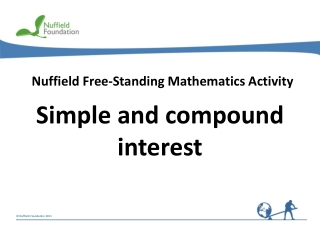DownloadDownload PresentationNuffield Free-Standing Mathematics Activity

# Nuffield Free-Standing Mathematics Activity

Download Presentation## Nuffield Free-Standing Mathematics Activity

- - - - - - - - - - - - - - - - - - - - - - - - - - - E N D - - - - - - - - - - - - - - - - - - - - - - - - - - -
##### Presentation Transcript

1. Nuffield Free-Standing Mathematics Activity Simple and compound interest

2. Where would be the best place to put the money you save? What do you need to consider when choosing a savings account? What are simple interest and compound interest? In this activity you will use a spreadsheet to compare how your savings would grow in accounts that pay simple interest with those that pay compound interest.

3. When choosing where to invest your savings, think about: • the interest rate you will get • how much money you can afford to save • how long you want to leave your money in the account • whether you can withdraw cash instantly, or have to give notice • Compare interest rates using the Annual Equivalent Rate (AER).

4. Source: http://www.moneysavingexpert.com/banking/interest-rates#compound

5. At the end of the activity • What are the differences in the way simple interest and compound interest are calculated? • What effect does this have on the amount of interest earned? • What difference does it make to the interest earned when you vary: • the amount invested • the rate of interest • the number of years?